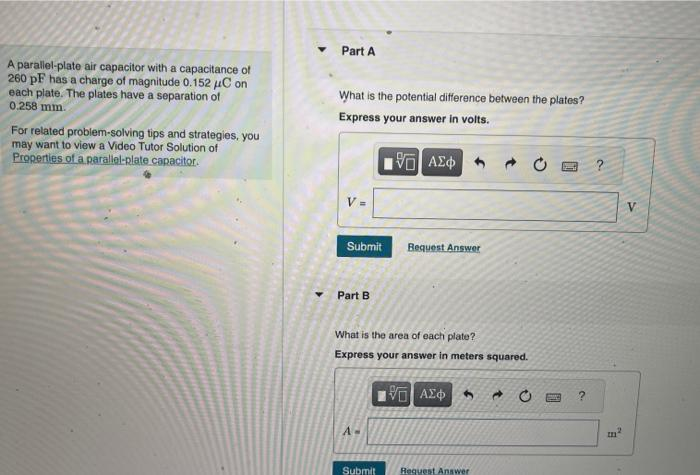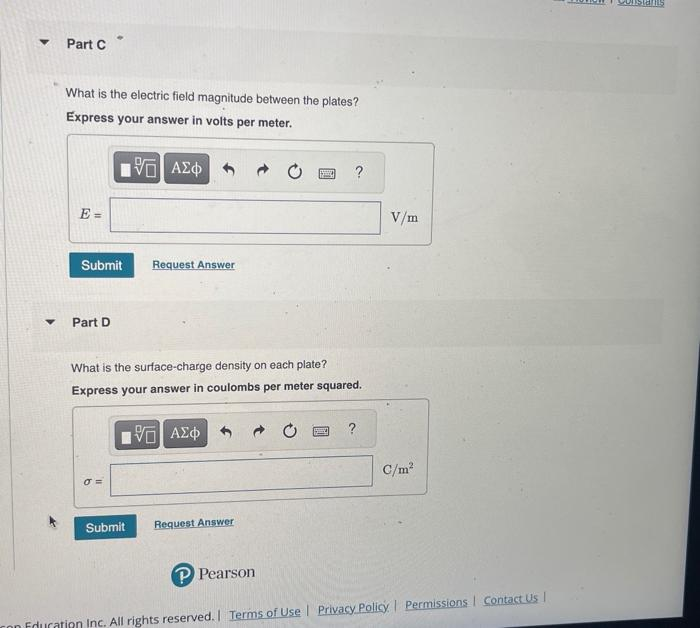Home / Expert Answers / Physics / a-parallel-plate-air-capacitor-with-a-capacitance-of-260pf-has-a-charge-of-magnitude-0-152c-on-ea-pa594

# (Solved): A parallel-plate air capacitor with a capacitance of 260pF has a charge of magnitude 0.152C on ea ...A parallel-plate air capacitor with a capacitance of has a charge of magnitude on each plate. The plates have a separation of . What is the potential difference between the plates? Express your answer in volts. For related problem-solving tips and strategies, you may want to view a Video Tutor Solution of Properties of a parallel-plate capacitor. Part B What is the area of each plate? Express your answer in meters squared. What is the electric field magnitude between the plates? Express your answer in volts per meter. Part D What is the surface-charge density on each plate? Express your answer in coulombs per meter squared.

We have an Answer from Expert